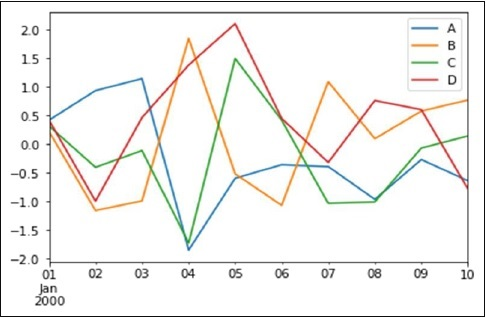# Pandas Built in Data Visualization | python crash course_05

## Pandas Built in Data Visualization:

Welcome to python crash course tutorial, today we will see the last topic Pandas Built in Data Visualization in the data science section.
SOME INTRODUCTION:
Data Visualizations is the presentation of data in graphical format. It help people understand the significance of data by summarizing and presenting a huge amount of data in a simple and easy-to-understand format and help communicate information clearly and effectively.
In this tutorial, we will learn about pandas built-in capabilities for data visualizations. It is built-off of matplotlib, but it baked into pandas for easier usage.
Let’s take a looks
Example:
Basic Plotting: plot

This functionality on Series and DataFrame is just a simple wrapper around the matplotlib libraries plot() methods.
```
import pandas as pd

import numpy as np

df = pd.DataFrame(np.random.randn(10,4),index=pd.date_range('1/1/2000',

periods=10), columns=list('ABCD'))

df.plot()
```
Output:If the index consists of dates, it calls gct().autofmt_xdate() to format the x-axis as shown in the above illustration.
We can plots one column versus another using the x and y keywords.
Plotting method allow a handful of plot styles other than the default line plot. These method can be provided as the kind keyword argument to plots(). These include −
1. bar or barh for bar plots
2. hist for histogram
3. box for boxplot
4. 'area' for area plots
5. 'scatter' for scatter plots

BEST OF LUCK!!!